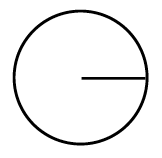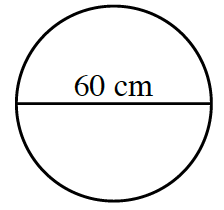### Home > CC3MN > Chapter 9 > Lesson 9.1.3 > Problem9-48

9-48.

Find the area of each circle below.

1.radius = $8$ cm

The formula to find the area of a circle is $r^{2}π$.

1.Remember to use the radius and not the diameter.

$π · (30 \text{ cm})^2 = 900π = 2827.43 \text{ cm}^2$